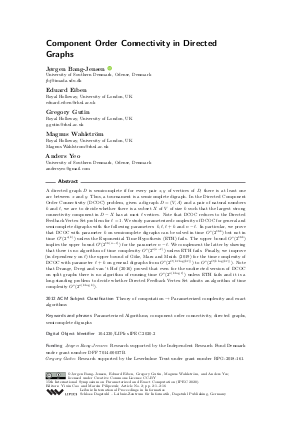Document# Component Order Connectivity in Directed Graphs

### Authors Jørgen Bang-Jensen, Eduard Eiben, Gregory Gutin, Magnus Wahlström, Anders Yeo## File

LIPIcs.IPEC.2020.2.pdf
• Filesize: 0.58 MB
• 16 pages

## Cite As

Jørgen Bang-Jensen, Eduard Eiben, Gregory Gutin, Magnus Wahlström, and Anders Yeo. Component Order Connectivity in Directed Graphs. In 15th International Symposium on Parameterized and Exact Computation (IPEC 2020). Leibniz International Proceedings in Informatics (LIPIcs), Volume 180, pp. 2:1-2:16, Schloss Dagstuhl - Leibniz-Zentrum für Informatik (2020)
https://doi.org/10.4230/LIPIcs.IPEC.2020.2

## Abstract

A directed graph D is semicomplete if for every pair x,y of vertices of D, there is at least one arc between x and y. Thus, a tournament is a semicomplete digraph. In the Directed Component Order Connectivity (DCOC) problem, given a digraph D = (V,A) and a pair of natural numbers k and 𝓁, we are to decide whether there is a subset X of V of size k such that the largest strong connectivity component in D-X has at most 𝓁 vertices. Note that DCOC reduces to the Directed Feedback Vertex Set problem for 𝓁 = 1. We study parameterized complexity of DCOC for general and semicomplete digraphs with the following parameters: k, 𝓁, 𝓁+k and n-𝓁. In particular, we prove that DCOC with parameter k on semicomplete digraphs can be solved in time O^*(2^(16k)) but not in time O^*(2^o(k)) unless the Exponential Time Hypothesis (ETH) fails. The upper bound O^*(2^(16k)) implies the upper bound O^*(2^(16(n-𝓁))) for the parameter n-𝓁. We complement the latter by showing that there is no algorithm of time complexity O^*(2^o(n-𝓁)) unless ETH fails. Finally, we improve (in dependency on 𝓁) the upper bound of Göke, Marx and Mnich (2019) for the time complexity of DCOC with parameter 𝓁+k on general digraphs from O^*(2^O(k𝓁 log (k𝓁))) to O^*(2^O(klog (k𝓁))). Note that Drange, Dregi and van 't Hof (2016) proved that even for the undirected version of DCOC on split graphs there is no algorithm of running time O^*(2^o(klog 𝓁)) unless ETH fails and it is a long-standing problem to decide whether Directed Feedback Vertex Set admits an algorithm of time complexity O^*(2^o(klog k)).

## Subject Classification

##### ACM Subject Classification
• Theory of computation → Parameterized complexity and exact algorithms
##### Keywords
• Parameterized Algorithms
• component order connectivity
• directed graphs
• semicomplete digraphs

## Metrics

• Access Statistics
• Total Accesses (updated on a weekly basis)
0

## References

1. J. Bang-Jensen and G. Gutin. Digraphs: Theory, Algorithms and Applications. Springer-Verlag, London, 2nd edition, 2009.2. J. Bang-Jensen and G.Z. Gutin, editors. Classes of Directed Graphs. Springer Monographs in Mathematics. Springer, 2018.3. J. Bang-Jensen and C. Thomassen. A polynomial algorithm for the 2-path problem for semicomplete digraphs. SIAM J. Discrete Math., 5(3):366-376, 1992. URL: https://doi.org/10.1137/0405027.
4. N.H. Bshouty and A. Gabizon. Almost optimal cover-free families. In Dimitris Fotakis, Aris Pagourtzis, and Vangelis Th. Paschos, editors, Algorithms and Complexity - 10th International Conference, CIAC 2017, Athens, Greece, May 24-26, 2017, Proceedings, volume 10236 of Lecture Notes in Computer Science, pages 140-151, 2017.5. Jonathan F. Buss and Judy Goldsmith. Nondeterminism within P. SIAM J. Comput., 22(3):560-572, 1993.6. Liming Cai and David Juedes. On the existence of subexponential parameterized algorithms. J. Comput. System Sci., 67(4):789-807, 2003.7. J. Chen, Y. Liu, S. Lu, B. O'Sullivan, and I. Razgon. A fixed-parameter algorithm for the directed feedback vertex set problem. J. Assoc. Comput. Mach., 55(5):21:1-21:19, 2008.8. M. Cygan, F.V. Fomin, L. Kowalik, D. Lokshtanov, D. Marx, M. Pilipczuk, M. Pilipczuk, and S. Saurabh. Parameterized Algorithms. Springer, 2015.9. R.G. Downey and M.R. Fellows. Parameterized Complexity. Monographs in Computer Science. Springer, 1999. URL: https://doi.org/10.1007/978-1-4612-0515-9.
10. R.G. Downey and M.R. Fellows. Fundamentals of Parameterized Complexity. Springer, 2013.11. P.G. Drange, M.S. Dregi, and P. van 't Hof. On the computational complexity of vertex integrity and component order connectivity. Algorithmica, 76(4):1181-1202, 2016.12. A. Göke, D. Marx, and M. Mnich. Parameterized algorithms for generalizations of directed feedback vertex set. In P. Heggernes, editor, Algorithms and Complexity. CIAC 2019, volume 11485 of Lecture Notes in Computer Science. Springer, Cham, 2019. URL: https://doi.org/10.1007/978-3-030-17402-6_21.
13. D. Gross, M. Heinig, L. Iswara, L. W. Kazmierczak, K. Luttrell, J. T. Saccoman, and C. Suffel. A survey of component order connectivity models of graph theoretic networks. WSEAS Transactions on Mathematics, 12:895-910, 2013.14. Russell Impagliazzo, Ramamohan Paturi, and Francis Zane. Which problems have strongly exponential complexity? J. Comput. System Sci., 63(4):512-530, 2001. Special issue on FOCS 98 (Palo Alto, CA).15. M. Kumar and D. Lokshtanov. A 2lk kernel for l-component order connectivity. In J. Guo and D. Hermelin, editors, 11th International Symposium on Parameterized and Exact Computation, IPEC 2016, August 24-26, 2016, Aarhus, Denmark, volume 63 of LIPIcs, pages 20:1-20:14. Schloss Dagstuhl - Leibniz-Zentrum fuer Informatik, 2016.16. E. Lee. Partitioning a graph into small pieces with applications to path transversal. Math. Program., 177(1-2):1-19, 2019.17. Rian Neogi, M. S. Ramanujan, Saket Saurabh, and Roohani Sharma. On the parameterized complexity of deletion to h-free strong components. In Javier Esparza and Daniel Král', editors, 45th International Symposium on Mathematical Foundations of Computer Science, MFCS 2020, August 24-28, 2020, Prague, Czech Republic, volume 170 of LIPIcs, pages 75:1-75:13. Schloss Dagstuhl - Leibniz-Zentrum für Informatik, 2020. URL: https://doi.org/10.4230/LIPIcs.MFCS.2020.75.
18. E. Speckenmeyer. On feedback problems in digraphs. In M. Nagl, editor, Graph-Theoretic Concepts in Computer Science, 15th International Workshop, WG '89, 1989, Proceedings, volume 411 of Lecture Notes in Computer Science, pages 218-231. Springer, 1989. URL: https://doi.org/10.1007/3-540-52292-1.
X

Feedback for Dagstuhl Publishing